Open in App
Not now

# Google Search Analysis with Python

• Last Updated : 27 Jun, 2022

In this article, we will be going through the approach to the analysis of google search in the python programming language.

Google does not share the exact number of searches, but it’s estimated that 228 million searches per hour or 5.8 billion searches per day are performed. That’s really a huge number! Let us do Google search analysis with the help of python based on search queries.

The article content:

• What is Pytrends?
• How to install Pytrends
• Interest Over Time
• Historical Hourly Interest
• Interest by Region
• Top Charts
• Related Queries
• Keyword Suggestion

## What is Pytrends?

Pytrends is an unofficial Google trends API used in python. It helps to analyze and list out the most popular Google search results on a specific topic or a subject, based on different regions and languages.

## How to install Pytrends?

To use this API, you first need to install it on your systems. You can easily install it using the command pip install pytrends.

`pip install pytrends`

Now, let’s get started with the task of analyzing the Google search trends by importing the required python libraries. First, we need to import pandas to create a dataframe. Second, we need to connect to Google as we are requesting the Google trending topics, so for this, we need to import the method TrendReq from pytrends.request library. Also, we will import matplotlib, to visualize the data.

## Python3

 `import` `pandas as pd ` `from` `pytrends.request ``import` `TrendReq ` `import` `matplotlib.pyplot as plt ` `Trending_topics ``=` `TrendReq(hl``=``'en-US'``, tz``=``360``)`

Now, we will be creating a dataframe of the top 10 countries that search for the term “CLOUD COMPUTING“. For this, we will be using the method build_payload, which allows storing a list of keywords that you want to search. In this, you can also specify the timeframe and the category to query the data from.

## Python3

 `kw_list``=``[``"Cloud Computing"``] ` `Trending_topics.build_payload(kw_list,cat``=``0``, timeframe``=``'today 12-m'``)`

## Interest Over Time

The interest_over_time() method, returns the historical, indexed data for when the specified keyword was most searched according to the timeframe mentioned in the build payload method.

## Python3

 `Trending_topics.build_payload(kw_list``=``[``"Cloud Computing"``], ` `                              ``cat``=``0``, timeframe``=``'today 12-m'``) ` `data ``=` `Trending_topics.interest_over_time() ` `data ``=` `data.sort_values(by``=``"Cloud Computing"``, ascending ``=` `False``) ` `data ``=` `data.head(``10``) ` `print``(data)`

Output: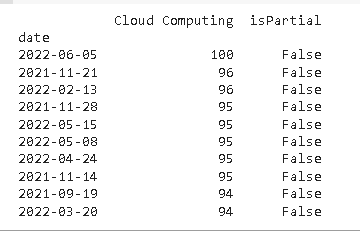## Historical Hour Interest

The get_historical_interest() method returns the historical, indexed, hourly data for when the specified keyword was most searched. You can also mention various time period parameters for which you want the historical data such as year_start, month_start, day_start, hour_start, year_end, month_end, day_end, and hour_end.

## Python3

 `kw_list ``=` `[``"Cloud Computing"``] ` `Trending_topics.build_payload(kw_list) ` `data ``=` `Trending_topics.get_historical_interest( ` `  ``kw_list, year_start``=``2018``, month_start``=``1``, day_start``=``1``, ` `  ``hour_start``=``0``, year_end``=``2018``, month_end``=``2``, day_end``=``1``, ` `  ``hour_end``=``0``, cat``=``0``, geo``=``'``', gprop='``', sleep``=``0``) ` `data ``=` `data.sort_values(by``=``"Cloud Computing"``, ascending ``=` `False``) ` `data ``=` `data.head(``10``) ` `print``(data)`

Output: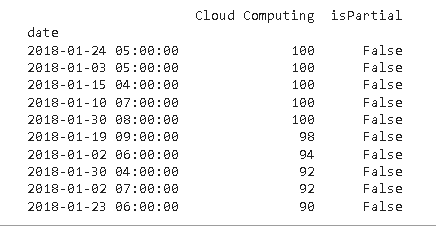## Interest By Region

Next is the interest_by_region method, this will let you know the performance of the keyword per region. It will show results on a scale of 0-100, where 100 indicates the country with the most search and 0 indicates with least search or not enough data.

## Python3

 `data ``=` `Trending_topics.interest_by_region() ` `data ``=` `data.sort_values(by``=``"Cloud Computing"``,  ` `                        ``ascending ``=` `False``) ` `data ``=` `data.head(``10``) ` `print``(data)`

After, running the above code you will get the output similar to the below output, depending on the timeframe mentioned in the build_payload method.

Output: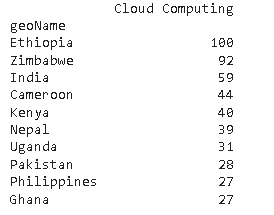Next, we can visualize the above data using a bar chart.

## Python3

 `data.reset_index().plot(x``=``'geoName'``, y``=``'Cloud Computing'``, ` `                        ``figsize``=``(``10``,``5``), kind``=``"bar"``) ` `plt.style.use(``'fivethirtyeight'``) ` `plt.show()`

Output: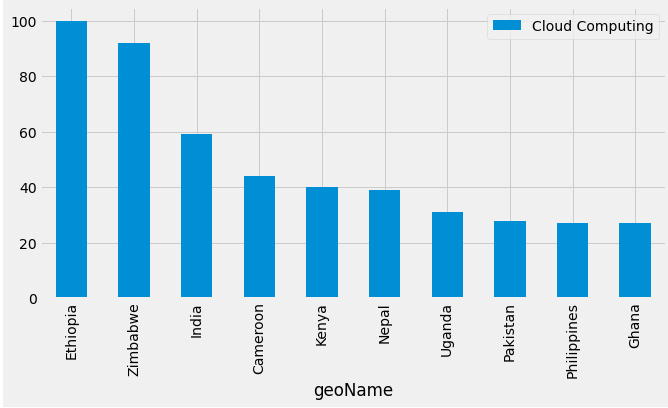## Top Charts

Using this method, we can get the top trending searches yearly. So, let us check what were the searches trending in the year 2020.

## Python3

 `df ``=` `Trending_topics.top_charts(``2020``, hl``=``'en-US'``,  ` `                                ``tz``=``300``, geo``=``'GLOBAL'``) ` `df.head(``10``) `

Output: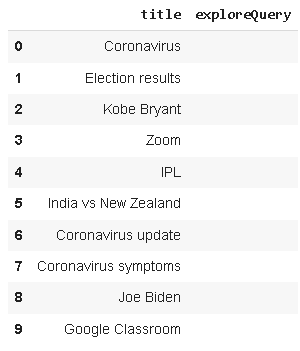From the above output, we can see, that the most searched topic of 2020 is “Coronavirus” and then the rest.

## Related Queries

Whenever a user searches for something about a particular topic on Google there is a high probability that the user will search for more queries related to the same topic. These are known as related queries. Let us find a list of related queries for “Cloud Computing”.

## Python3

 `Trending_topics.build_payload(kw_list``=``[``'Cloud Computing'``]) ` `related_queries ``=` `Trending_topics.related_queries() ` `related_queries.values()`

Below are some of the queries mostly searched on Google related to Cloud Computing.

Output: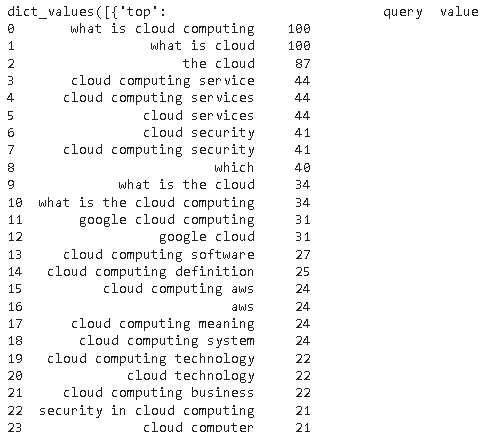## Keyword Suggestions

The suggestions() method, will help you to explore what the world is searching for. It returns a list of additional suggested keywords that can be used to filter a trending search on Google.

## Python3

 `keywords ``=` `Trending_topics.suggestions( ` `  ``keyword``=``'Cloud Computing'``) ` `df ``=` `pd.DataFrame(keywords) ` `df.drop(columns``=` `'mid'``)  `

Output: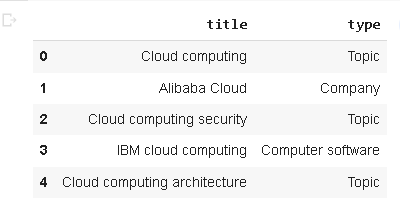My Personal Notes arrow_drop_up
Related Articles